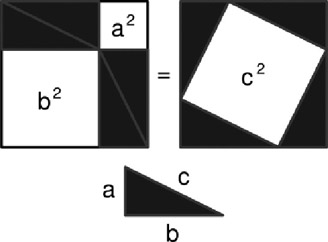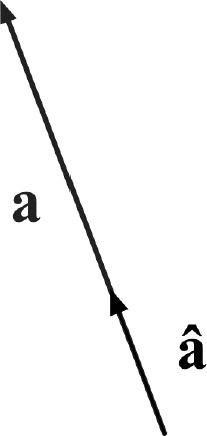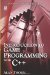# 3.13 Vectors

## 3.13 Vectors

On paper, vectors look exactly the same as Cartesian coordinates, as both have an x and a y component. However, vertices represent points in space, while vectors represent direction. A vector simply points in a direction, indicating where something must travel. Vectors are useful for games because they can indicate the direction in which objects are moving.Figure 3.26

### 3.13.1 Vector Magnitude

Magnitude can describe the size of an angle, but here it refers to the length of a vector; that is, the linear distance along the diagonal. Calculating the length of a vector is simple; it is the Pythagorean theorem.Figure 3.27

### 3.13.2 Unit Vector

A vector is said to be normalized when it is a unit vector. A unit vector is a vector whose magnitude is 1. It has no length and indicates only direction.Figure 3.28Introduction to Game Programming with C++ (Wordware Game Developers Library)
ISBN: 1598220322
EAN: 2147483647
Year: 2007
Pages: 225
Authors: Alan Thorn

Similar book on Amazon

flylib.com © 2008-2017.
If you may any questions please contact us: flylib@qtcs.net